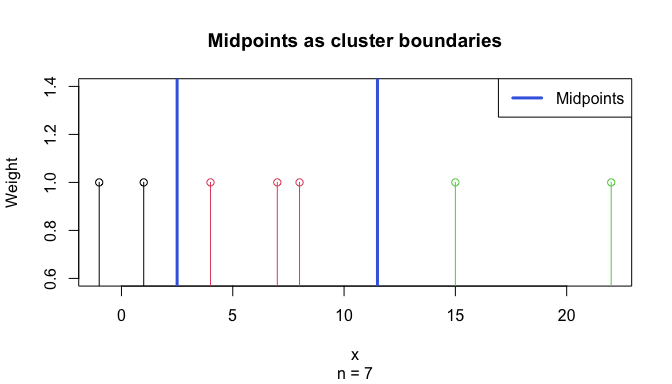# Tutorial: Optimal univariate clustering

#### Updated 2020-01-20

This tutorial illustrates applications of optimal univariate clustering function Ckmeans.1d.dp. It clusters univariate data given the number of clusters $$k$$. It can estimate $$k$$ if not provided. It can also perform optimal weighted clustering when a weight vector is provided with the input univariate data. Weighted clustering can be used to analyze 1-D signals such as time series data. The corresponding clusters obtained from weighted clustering can be the basis for optimal time course segmentation or optimal peak calling.

## 1. Cluster univariate data given the number of clusters $$k$$

### Example 1.

Cluster data generated from a Gaussian mixture model of three components.

The number of clusters is provided.

require(Ckmeans.1d.dp)
## Loading required package: Ckmeans.1d.dp
x <- c(rnorm(50, sd=0.3), rnorm(50, mean=1, sd=0.3), rnorm(50, mean=2, sd=0.3))
# Divide x into 3 clusters
k <- 3
result <- Ckmeans.1d.dp(x, k)
plot(result)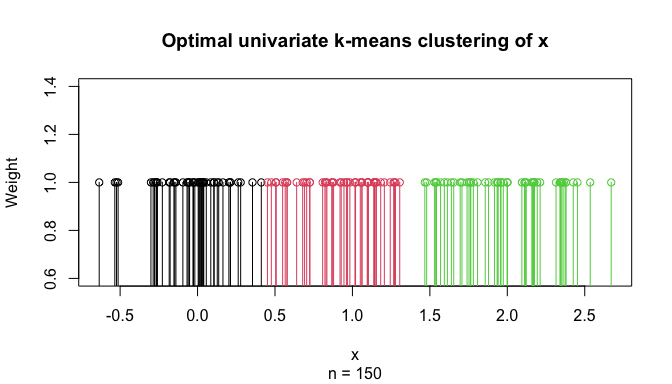plot(x, col=result$cluster, pch=result$cluster, cex=1.5,
main="Optimal univariate clustering given k",
sub=paste("Number of clusters given:", k))
abline(h=result$centers, col=1:k, lty="dashed", lwd=2) legend("bottomright", paste("Cluster", 1:k), col=1:k, pch=1:k, cex=1.5, bty="n")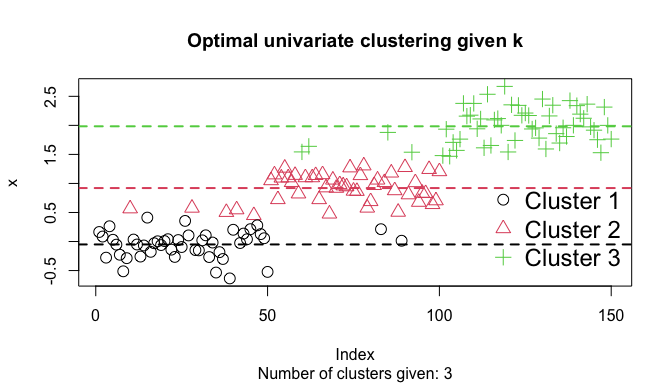## 2. Cluster univariate data with an estimated number of clusters $$k$$ ### Example 2. Cluster data generated from a Gaussian mixture model of three components. The number of clusters is determined by Bayesian information criterion: require(Ckmeans.1d.dp) x <- c(rnorm(50, mean=-1, sd=0.3), rnorm(50, mean=1, sd=1), rnorm(50, mean=2, sd=0.4)) # Divide x into k clusters, k automatically selected (default: 1~9) result <- Ckmeans.1d.dp(x) plot(result)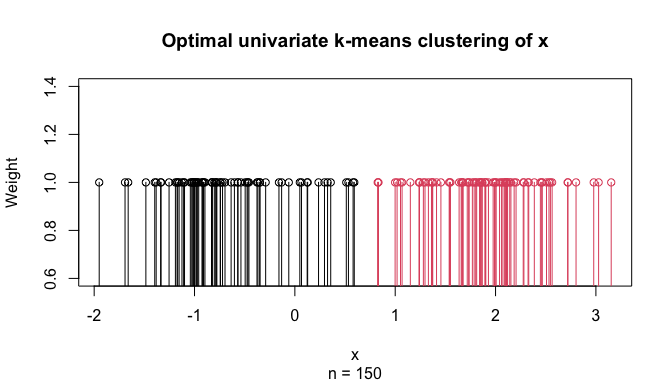k <- max(result$cluster)
plot(x, col=result$cluster, pch=result$cluster, cex=1.5,
main="Optimal univariate clustering with k estimated",
sub=paste("Number of clusters is estimated to be", k))
text(res$centers, max(w) * .95, cex=0.75, font=2, paste(round(res$size / sum(res$size) * 100), "/ 100"))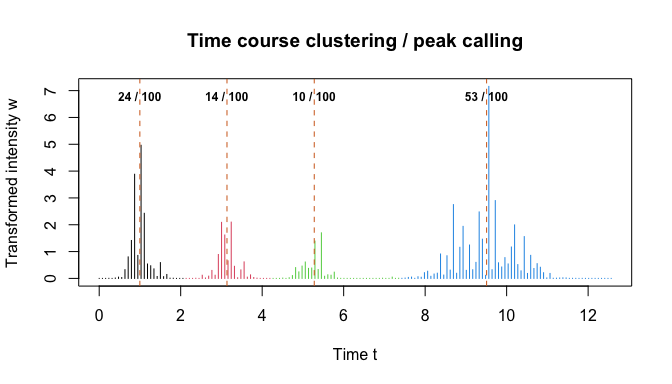## 4. Obtaining midpoints between consecutive clusters It is often desirable to visualize boundaries between consecutive clusters. The ahist() function offers several ways to estimate cluster boundaries. The simplest is to use the midpoint between the two closest points in two consecutive clusters, as illustrated in the code below. x <- c(7, 4, 1, 8, 15, 22, -1) k <- 3 ckm <- Ckmeans.1d.dp(x, k=k) midpoints <- ahist(ckm, style="midpoints", data=x, plot=FALSE)$breaks[2:k]

plot(ckm, main="Midpoints as cluster boundaries")
abline(v=midpoints, col="RoyalBlue", lwd=3)
legend("topright", "Midpoints", lwd=3, col="RoyalBlue")